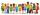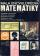Stairs

Between adjacent levels are 15 stairs. If step will be 1.2 cm lower there would be 16 stairs. What is the stair height?

Result

x =  19.2 cm

Solution:

15x = 16(x-1.2)

x = 19.2

x = 965 = 19.2

Calculated by our simple equation calculator.

Leave us a comment of example and its solution (i.e. if it is still somewhat unclear...):Be the first to comment!To solve this verbal math problem are needed these knowledge from mathematics:

Do you have a linear equation or system of equations and looking for its solution? Or do you have quadratic equation? Do you want to convert length units?

Next similar examples:

1. NormThree workers planted 3555 seedlings of tomatoes in one dey. First worked at the standard norm, the second planted 120 seedlings more and the third 135 seedlings more than the first worker. How many seedlings were standard norm?
2. Tickets 3A total of 645 tickets were sold for the school play. They were either adult tickets or student tickets. There were 55 fewer student tickets sold than adult tickets. How many adult tickets were sold?
3. One-thirdA one-third of unknown number is equal to five times as great as the difference of the same unknown number and number 28. Determine the unknown number.
4. Equation?
5. QuizTested student answered correctly on a 4/ 8 questions. Wrong answers was 16. How many questions answered?
6. InfirmaryTwo thirds of children from the infirmary went on a trip seventh went to bathe and 40 children remained in the gym. How many children were treated in the infirmary?
7. The classroomIn the seventh class the number of boys and girls are at a ratio of 4:5. Boys are 16. How many total students are in the classroom?
8. Dropped sheetsThree consecutive sheets dropped from the book. The sum of the numbers on the pages of the dropped sheets is 273. What number has the last page of the dropped sheets?
9. Equation 29Solve next equation: 2 ( 2x + 3 ) = 8 ( 1 - x) -5 ( x -2 )
10. Find xSolve: if 2(x-1)=14, then x= (solve an equation with one unknown)
11. BookTo number pages of thick book was used 4201 digits. How many pages has this book?
12. Negative in equation2x + 3 + 7x = – 24, what is the value of x?
13. Unknown number 11That number increased by three equals three times itself?
14. 1.5 divided1.5 divided by 1 = w divided by 4
15. FactoryIn the factory workers work in three shifts. In the first inning operates half of all employees in the second inning and a third in the third inning 200 employees. How many employees work at the factory?
16. Simple equationSolve the following simple equation: 2. (4x + 3) = 2-5. (1-x)
17. Unknown numberIdentify unknown number which 1/5 is 40 greater than one tenth of that number.